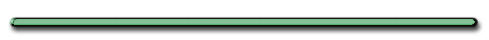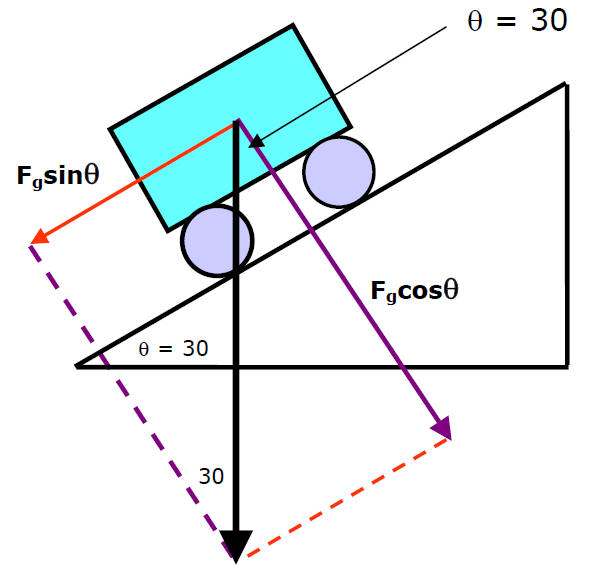Resolving Weight
force on Ramp

Parallel & perpendicular components

St. Mary's H.S. PhysicsF = 10. N (Weight)Fn = Fgcosq

Fn = mgcosqFind f if box is stationaryFnet = 0

f = Fgsinq =  mgsinqBox at rest, Find Usf = us[Fgcosq] = Fgsinq

Us = tanqFinding a horizontal component

&** AP - Ramp**